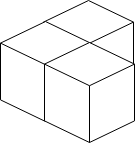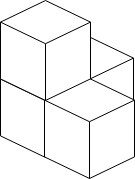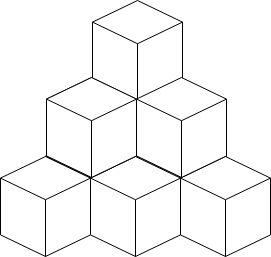# GeetCode Hub

You have a cubic storeroom where the width, length, and height of the room are all equal to `n` units. You are asked to place `n` boxes in this room where each box is a cube of unit side length. There are however some rules to placing the boxes:

• You can place the boxes anywhere on the floor.
• If box `x` is placed on top of the box `y`, then each side of the four vertical sides of the box `y` must either be adjacent to another box or to a wall.

Given an integer `n`, return the minimum possible number of boxes touching the floor.

Example 1:```Input: n = 3
Output: 3
Explanation: The figure above is for the placement of the three boxes.
These boxes are placed in the corner of the room, where the corner is on the left side.
```

Example 2:```Input: n = 4
Output: 3
Explanation: The figure above is for the placement of the four boxes.
These boxes are placed in the corner of the room, where the corner is on the left side.
```

Example 3:```Input: n = 10
Output: 6
Explanation: The figure above is for the placement of the ten boxes.
These boxes are placed in the corner of the room, where the corner is on the back side.```

Constraints:

• `1 <= n <= 109`

class Solution { public int minimumBoxes(int n) { } }